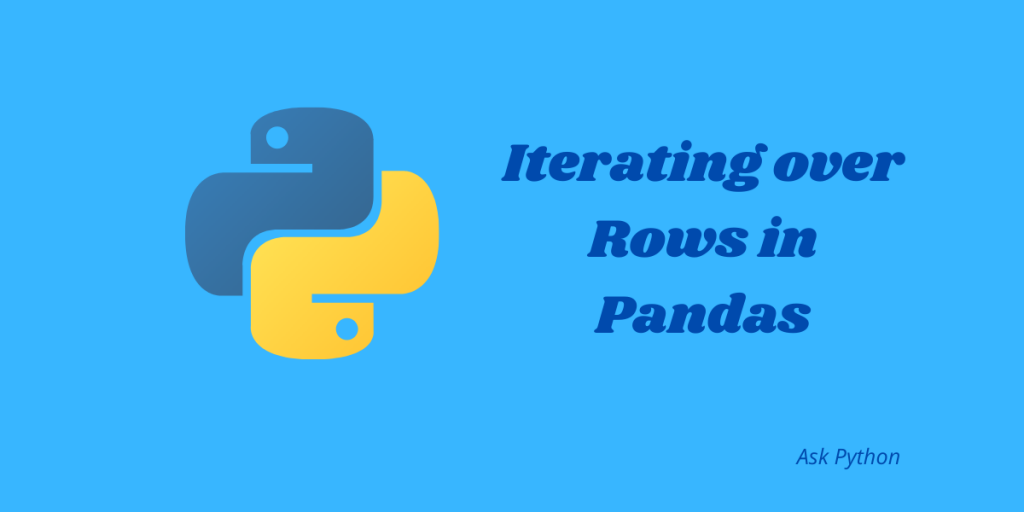# How to Iterate Over Rows in Pandas Dataframe?The word iteration means the process of taking each of the elements contained in a data structure one after another. In python, we use loops to go over items a number of times. We can also term iteration as “ repetitive execution of items”. Pandas is an extremely useful library in Python as it provides a number of tools for data analysis. In this article, we will learn how we can iterate over rows in a Pandas DataFrame. So let’s get started!

## What is the Pandas DataFrame?

Pandas DataFrame is a two-dimensional tabular data structure consisting of rows and columns. DataFrame is a mutable data structure in Python.

For example:

```import pandas as pd

#Creating the data
data = {'Name':['Tommy','Linda','Justin','Brendon'], 'Marks':[100,200,300,600]}
df= pd.DataFrame(data)
print(df)
```

Output:

```      Name        Marks
0    Tommy    100
1    Linda       200
2   Justin       300
3  Brendon    600
```

Now let’s look at the methods for iterating over rows.

## Methods to iterate over rows in Pandas DataFrame

There are many methods that you can apply to iterate over rows in a Pandas DataFrame but each method comes with its own advantages and disadvantages.

### 1. Using iterrows() method

This is one of the simple and straightforward methods to iterate over rows in Python. Although it is the most simple method, the iteration takes place slowly and is not much efficient. This method will return the entire row along with the row index.

For example:

```import pandas as pd

data = {'Name': ['Tommy', 'Linda', 'Justin', 'Brendon'],
'Age': [21, 19, 20, 18],
'Subject': ['Math', 'Commerce', 'Arts', 'Biology'],
'Scores': [88, 92, 95, 70]}

df = pd.DataFrame(data, columns = ['Name', 'Age', 'Subject', 'Scores'])

print("The DataFrame is :\n", df)

print("\nPerforming Interation using iterrows() method :\n")

# iterate through each row and select 'Name' and 'Scores' column respectively.
for index, row in df.iterrows():
print (row["Name"], row["Scores"])
```

Output:

```The DataFrame is :
Name  Age   Subject  Scores
0    Tommy   21      Math      88
1    Linda   19  Commerce      92
2   Justin   20      Arts      95
3  Brendon   18   Biology      70

Performing Interation using iterrows() method :

Tommy 88
Linda 92
Justin 95
Brendon 70
```

### 2. Using the itertuples() method

This method is very much similar to the iterrows() method except for the fact that it returns named tuples. With the help of tuples, you can access the specific values as an attribute, or in other words, we can access very specific values of a row in a column. This is a much more robust method and the iteration takes place at a faster rate than the iterrows() method.

For example:

```import pandas as pd

# Creating a dictionary containing students data
data = {'Name': ['Tommy', 'Linda', 'Justin', 'Brendon'],
'Age': [21, 19, 20, 18],
'Subject': ['Math', 'Commerce', 'Arts', 'Biology'],
'Scores': [88, 92, 95, 70]}

# Converting the dictionary into DataFrame
df = pd.DataFrame(data, columns = ['Name', 'Age', 'Subject', 'Scores'])

print("Given Dataframe :\n", df)

print("\n Performing iteration over rows using itertuples() method :\n")

# iterate through each row and select 'Name' and 'Scores' column respectively.
for row in df.itertuples(index = True, name ='Pandas'):
print (getattr(row, "Name"), getattr(row, "Scores"))
```

Output:

```Given Dataframe :
Name  Age   Subject  Scores
0    Tommy   21      Math      88
1    Linda   19  Commerce      92
2   Justin   20      Arts      95
3  Brendon   18   Biology      70

Performing iteration over rows using itertuples() method :

Tommy 88
Linda 92
Justin 95
Brendon 70
```

### 3. Using the apply () method

This method is the most efficient method and has faster runtimes than the above two methods.

For example:

```import pandas as pd
import pandas as pd

# Creating a dictionary containing students data
data = {'Name': ['Tommy', 'Linda', 'Justin', 'Brendon'],
'Age': [21, 19, 20, 18],
'Subject': ['Math', 'Commerce', 'Arts', 'Biology'],
'Scores': [88, 92, 95, 70]}

# Converting the dictionary into DataFrame
df = pd.DataFrame(data, columns = ['Name', 'Age', 'Stream', 'Scores'])

print("Given Dataframe :\n", df)

print("\nPerforming Iteration over rows using apply function :\n")

# iterate through each row and concatenate 'Name' and 'Scores' column
print(df.apply(lambda row: row["Name"] + " " + str(row["Scores"]), axis = 1))
```

Output:

```Given Dataframe :
Name  Age Stream  Scores
0    Tommy   21    NaN      88
1    Linda   19    NaN      92
2   Justin   20    NaN      95
3  Brendon   18    NaN      70

Performing Iteration over rows using apply function :

0      Tommy 88
1      Linda 92
2     Justin 95
3    Brendon 70
dtype: object
```

### 4. Using the iloc [] function

This is yet another simple function we can use to iterate over rows. We will select the index of the columns after iteration using the iloc[] function.

For example:

```import pandas as pd

# Creating a dictionary containing students data
data = {'Name': ['Tommy', 'Linda', 'Justin', 'Brendon'],
'Age': [21, 19, 20, 18],
'Subject': ['Math', 'Commerce', 'Arts', 'Biology'],
'Scores': [88, 92, 95, 70]}

# Converting the dictionary into DataFrame
df = pd.DataFrame(data, columns = ['Name', 'Age', 'Subject', 'Scores'])

print("Given Dataframe :\n", df)

print("\nIterating over rows using iloc function :\n")

# iterate through each row and select 0th and 3rd index column
for i in range(len(df)) :
print(df.iloc[i, 0], df.iloc[i, 3])
```

Output:

```Given Dataframe :
Name  Age   Subject  Scores
0    Tommy   21      Math      88
1    Linda   19  Commerce      92
2   Justin   20      Arts      95
3  Brendon   18   Biology      70

Performing Iteration over rows using iloc function :

Tommy 88
Linda 92
Justin 95
Brendon 70

```

## Conclusion

In this article, we learned different methods to iterate over rows in python. iterrows() and itertuples() method are not the most efficient method to iterate over DataFrame rows though they are fairly simple. For better results and faster runtimes, you should look for the apply() method.